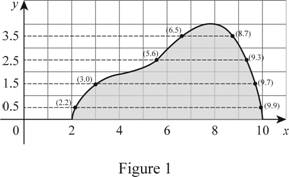# The volume of the solid region rotated about the x -axis using the midpoint rule.### Single Variable Calculus: Concepts...

4th Edition
James Stewart
Publisher: Cengage Learning
ISBN: 9781337687805### Single Variable Calculus: Concepts...

4th Edition
James Stewart
Publisher: Cengage Learning
ISBN: 9781337687805

#### Solutions

Chapter 6.2, Problem 29E

a)

To determine

## To calculate: The volume of the solid region rotated about the x-axis using the midpoint rule.

Expert Solution

The volume of the solid region rotated about the x-axis is 195.66.

### Explanation of Solution

Given information:

Number of intervals abf(x)dxi=1nf(x¯i)Δx=Δx(f(x¯1)+.......+f(x¯n)).

The region lies between Δx=ban and x¯i=12(xi1+xi).

The endpoints are, 2, 4, 6, 8, and 10.

The midpoints are, 3, 5, 7, and 9.

Apply midpoint rule:

Δx

Where, (xi1,xi) and x¯i

Here, width of the subintervals is n=4, the upper limit is b, the lower limit is a and the midpoint of a=2 is b=10.

Calculation:

The expression to find the volume as shown below.

V=abA(x)dx (1)

The expression to find the volume of the solid region using midpoint rule as shown below.

abA(x)dx=ban(A(3)+A(5)+A(7)+A(9))=ban(π(f(3))2+π(f(5))2+π(f(7))2+π(f(9))2) (2)

Substitute 2 for a, 10 m for b, 4 for n, 1.5 for f(3), 2.2 for f(5), 3.8 for f(7), and 3.1 for f(9) in Equation (2).

abA(x)dx=1024((π×1.52)+(π×2.22)+(π×3.82)+(π×3.12))=2π×(2.25+4.84+14.44+9.61)=2π×31.91=195.66

Therefore, the volume of the solid region rotated about the x-axis is 195.66.

b)

To determine

### To calculate: The volume of the solid region rotated about the y-axis using the midpoint rule.

Expert Solution

The volume of the solid region rotated about the y-axis is 838.27.

### Explanation of Solution

Given information:

Number of intervals n=4.

The region lies between a=0 and b=4.

The endpoints are, 0, 1, 2, 3 and 4.

The midpoints are, 0.5, 1.5, 2.5, and 3.5.

Calculation:

The expression to find the volume of the solid region using midpoint rule as shown below.

abA(x)dx=ban(A(0.5)+A(1.5)+A(2.5)+A(3.5))=ban(π(rout2rin2)0.5+π(rout2rin2)1.5+π(rout2rin2)2.5+π(rout2rin2)3.5) (3)

Sketch the region as shown in Figure 1.Refer to Figure 1.

At the midpoint 0.5.

rout=9.9rin=2.2

At the midpoint 1.5.

rout=9.7rin=3.0

At the midpoint 2.5.

rout=9.3rin=5.6

At the midpoint 3.5.

rout=8.7rin=6.5

Substitute 0 for a, 4 for b, 4 for n, and substitute the known values in Equation (4).

abA(x)dx=404×π[(9.922.22)+(9.723.02)+(9.325.62)+(8.726.52)]=π×(93.17+85.09+55.13+33.44)=π×266.83=838.27

Therefore, the volume of the solid region rotated about the y-axis is 838.27.

### Have a homework question?

Subscribe to bartleby learn! Ask subject matter experts 30 homework questions each month. Plus, you’ll have access to millions of step-by-step textbook answers!